Скачать презентацию PROFIT LOSS Department of Analytical Skills

4759700770df62c9032bcce83a06ed20.ppt

• Количество слайдов: 45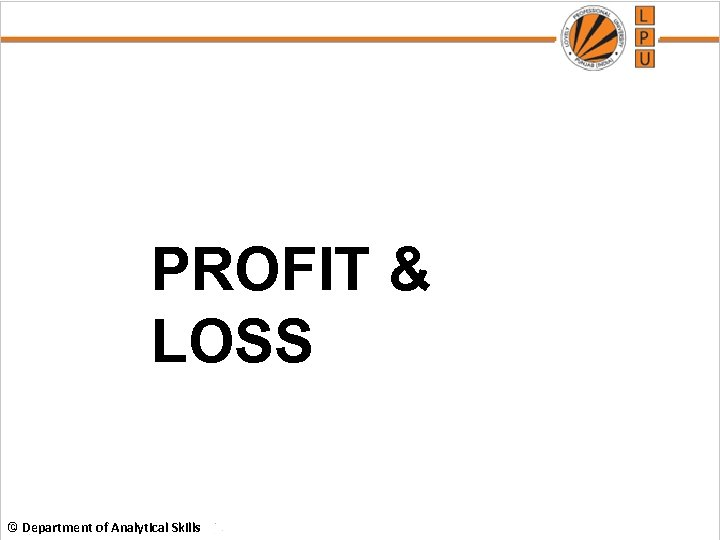PROFIT & LOSS © Department of Analytical Skills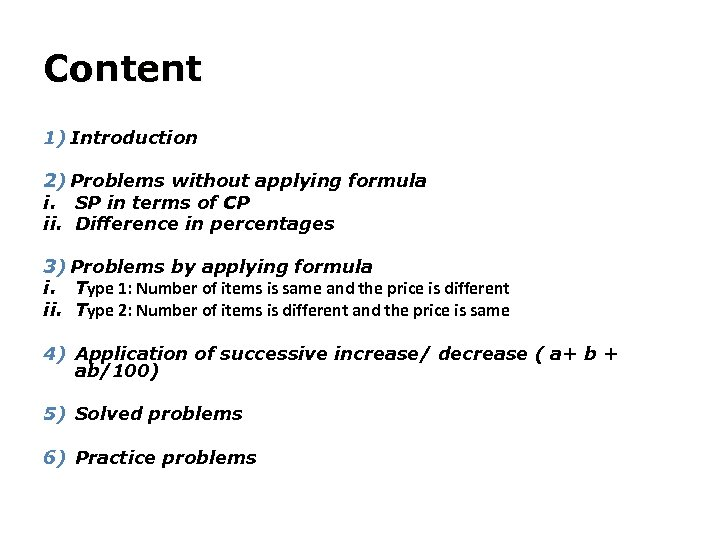Content 1) Introduction 2) Problems without applying formula i. SP in terms of CP ii. Difference in percentages 3) Problems by applying formula i. Type 1: Number of items is same and the price is different ii. Type 2: Number of items is different and the price is same 4) Application of successive increase/ decrease ( a+ b + ab/100) 5) Solved problems 6) Practice problems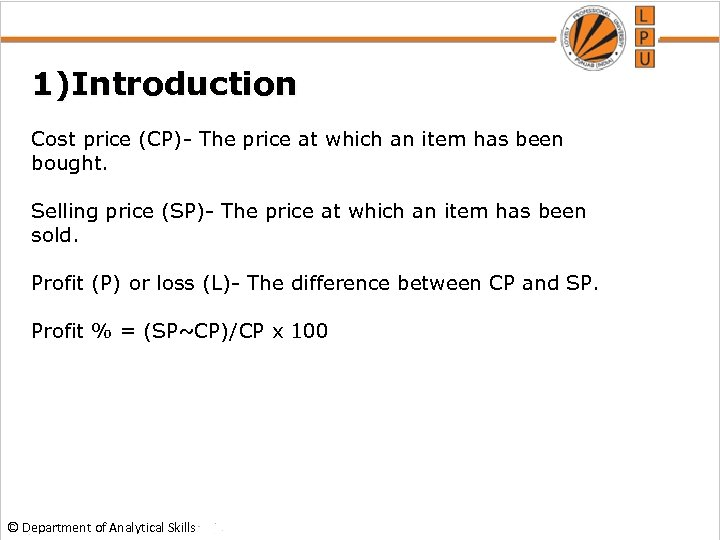1)Introduction Cost price (CP)- The price at which an item has been bought. Selling price (SP)- The price at which an item has been sold. ` Profit (P) or loss (L)- The difference between CP and SP. Profit % = (SP~CP)/CP x 100 © Department of Analytical Skills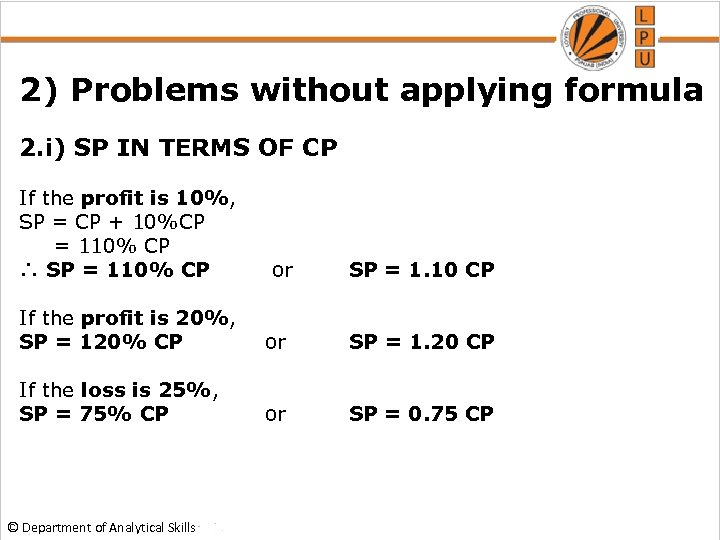2) Problems without applying formula 2. i) SP IN TERMS OF CP If the profit is 10%, SP = CP + 10%CP = 110% CP ∴ SP = 110% CP or SP = 1. 10 CP If the profit is 20%, SP = 120% CP or SP = 1. 20 CP If the loss is 25%, SP = 75% CP or SP = 0. 75 CP © Department of Analytical Skills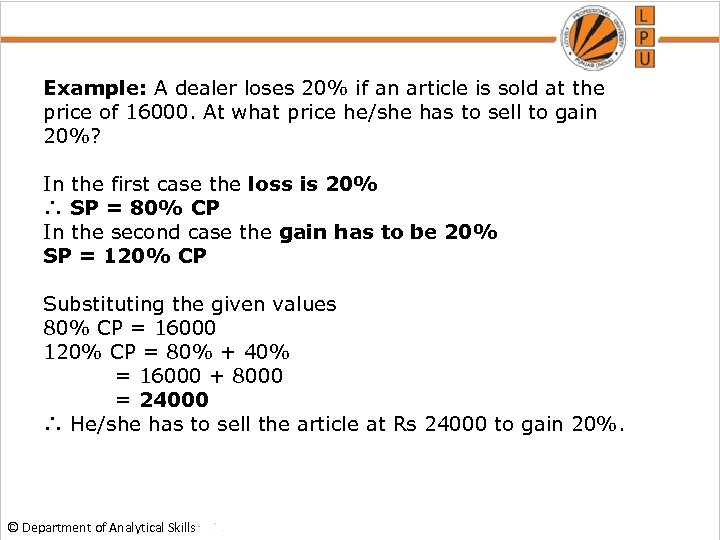Example: A dealer loses 20% if an article is sold at the price of 16000. At what price he/she has to sell to gain 20%? In the first case the loss is 20% ∴ SP = 80% CP In the second case the gain has to be 20% SP = 120% CP Substituting the given values 80% CP = 16000 120% CP = 80% + 40% = 16000 + 8000 = 24000 ∴ He/she has to sell the article at Rs 24000 to gain 20%. © Department of Analytical Skills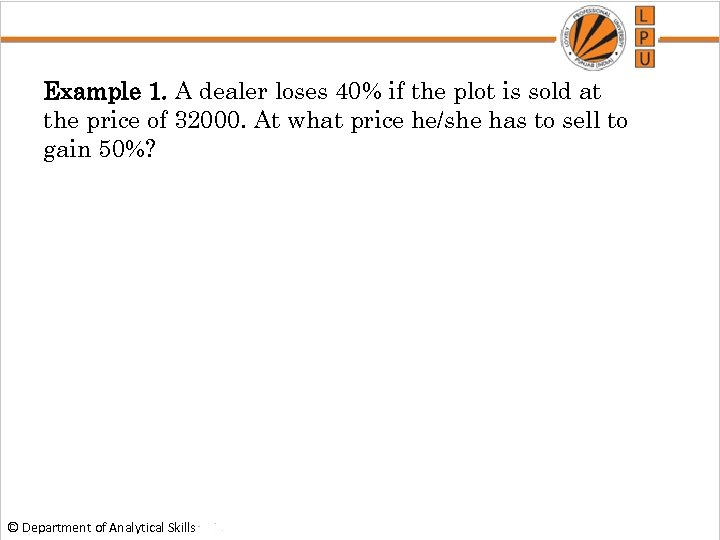Example 1. A dealer loses 40% if the plot is sold at the price of 32000. At what price he/she has to sell to gain 50%? © Department of Analytical Skills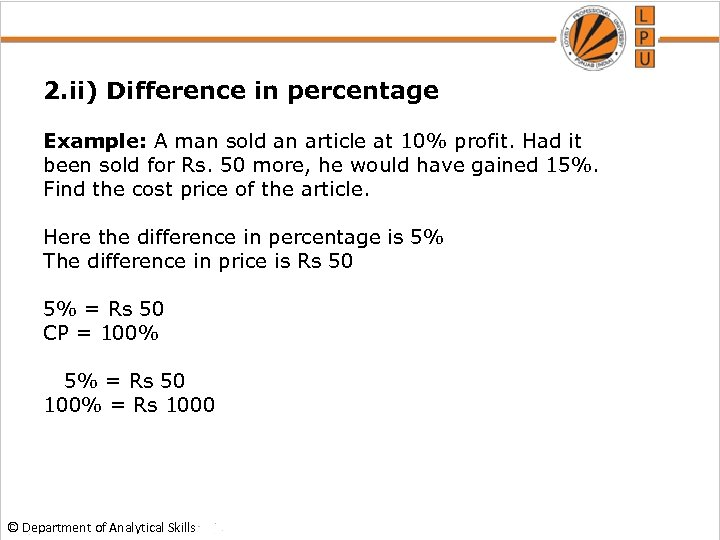2. ii) Difference in percentage Example: A man sold an article at 10% profit. Had it been sold for Rs. 50 more, he would have gained 15%. Find the cost price of the article. Here the difference in percentage is 5% The difference in price is Rs 50 5% = Rs 50 CP = 100% 5% = Rs 50 100% = Rs 1000 © Department of Analytical Skills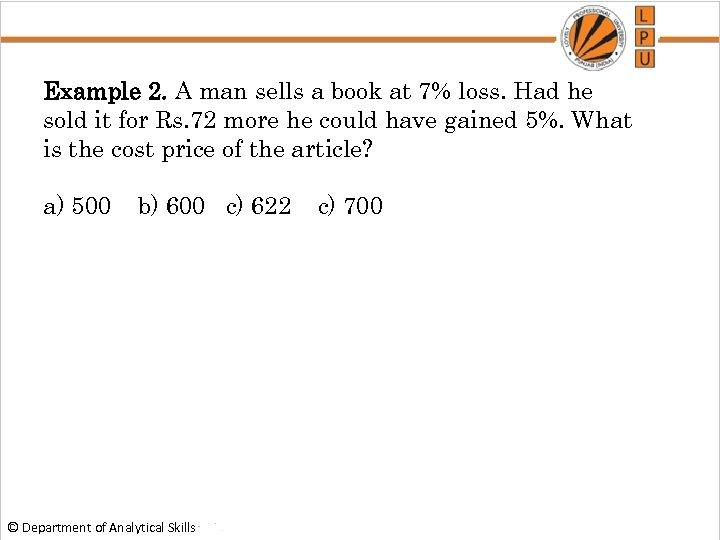Example 2. A man sells a book at 7% loss. Had he sold it for Rs. 72 more he could have gained 5%. What is the cost price of the article? a) 500 b) 600 c) 622 c) 700 © Department of Analytical Skills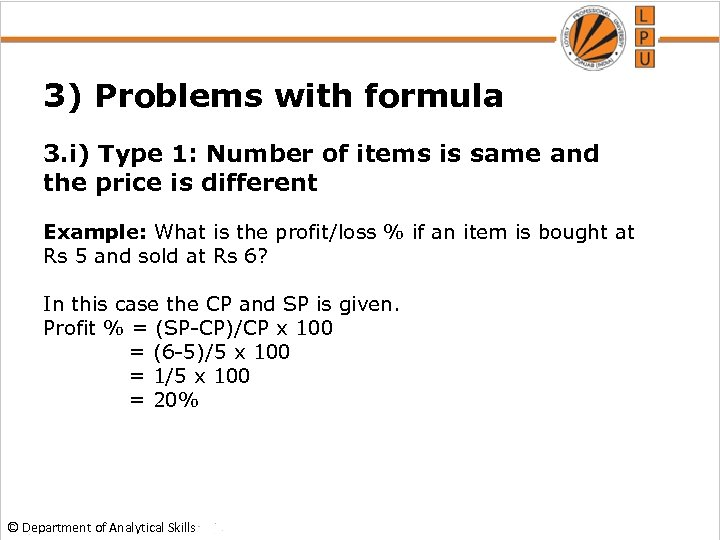3) Problems with formula 3. i) Type 1: Number of items is same and the price is different Example: What is the profit/loss % if an item is bought at Rs 5 and sold at Rs 6? In this case the CP and SP is given. Profit % = (SP-CP)/CP x 100 = (6 -5)/5 x 100 = 1/5 x 100 = 20% © Department of Analytical Skills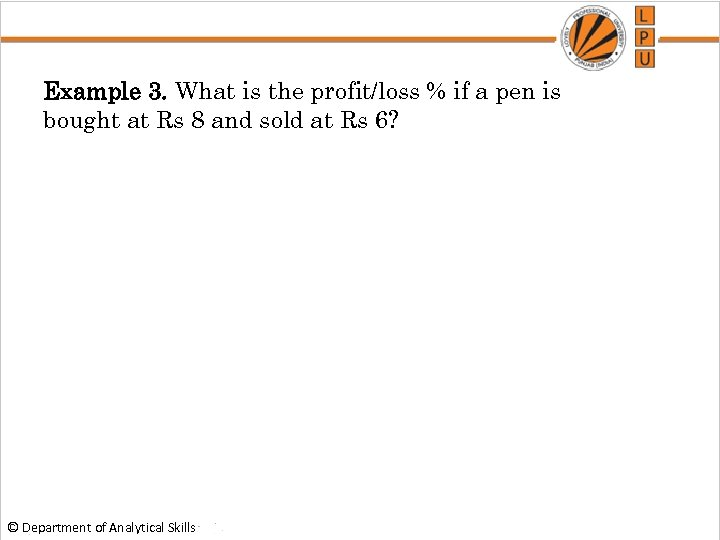Example 3. What is the profit/loss % if a pen is bought at Rs 8 and sold at Rs 6? © Department of Analytical Skills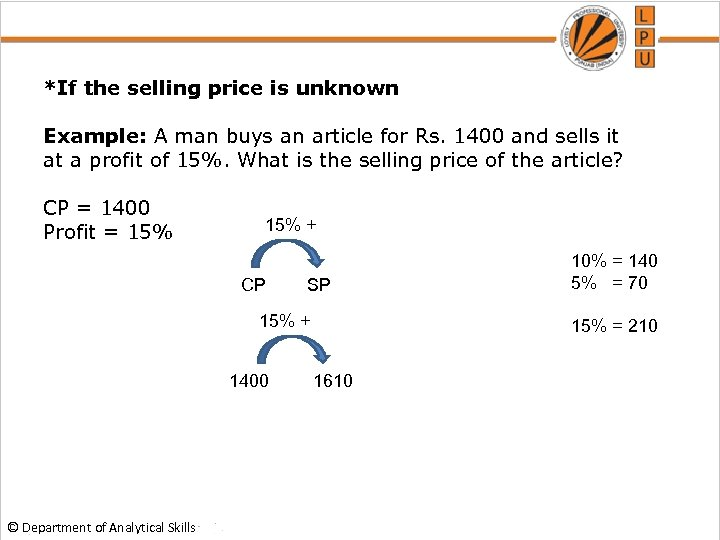*If the selling price is unknown Example: A man buys an article for Rs. 1400 and sells it at a profit of 15%. What is the selling price of the article? CP = 1400 Profit = 15% + CP SP 15% + 1400 © Department of Analytical Skills 10% = 140 5% = 70 15% = 210 1610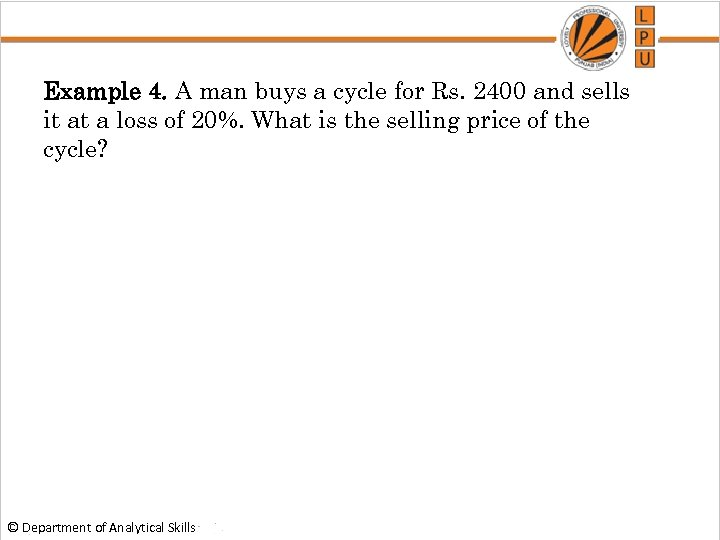Example 4. A man buys a cycle for Rs. 2400 and sells it at a loss of 20%. What is the selling price of the cycle? © Department of Analytical Skills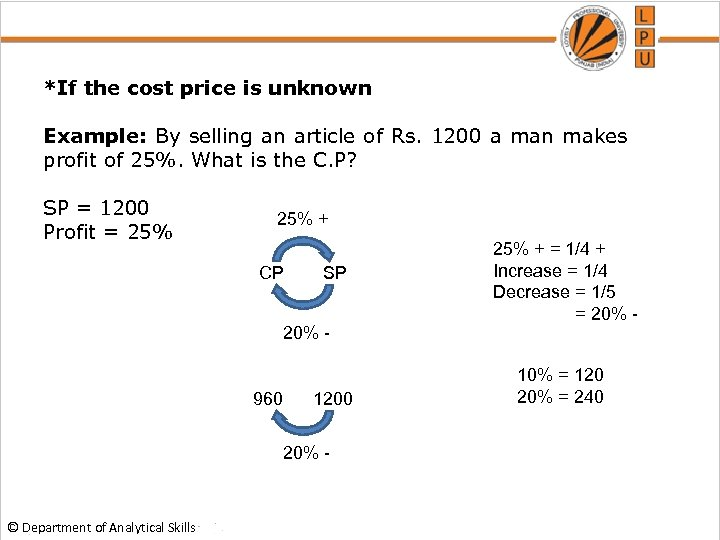*If the cost price is unknown Example: By selling an article of Rs. 1200 a man makes profit of 25%. What is the C. P? SP = 1200 Profit = 25% + CP SP 20% - 960 1200 20% - © Department of Analytical Skills 25% + = 1/4 + Increase = 1/4 Decrease = 1/5 = 20% 10% = 120 20% = 240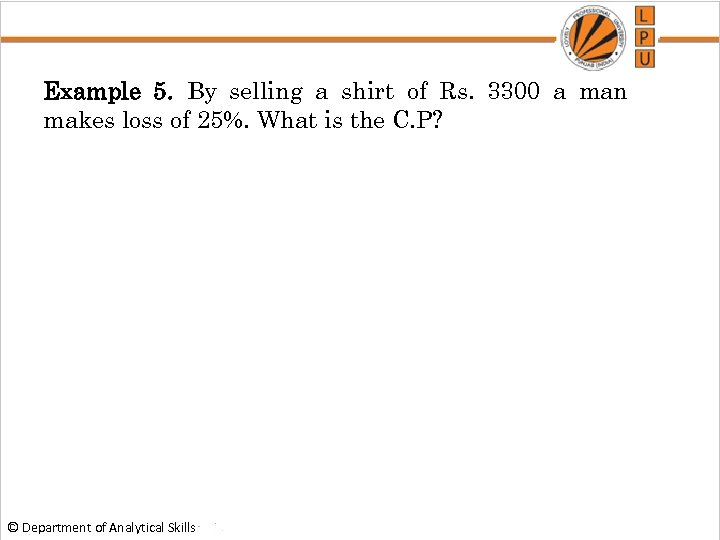Example 5. By selling a shirt of Rs. 3300 a man makes loss of 25%. What is the C. P? © Department of Analytical Skills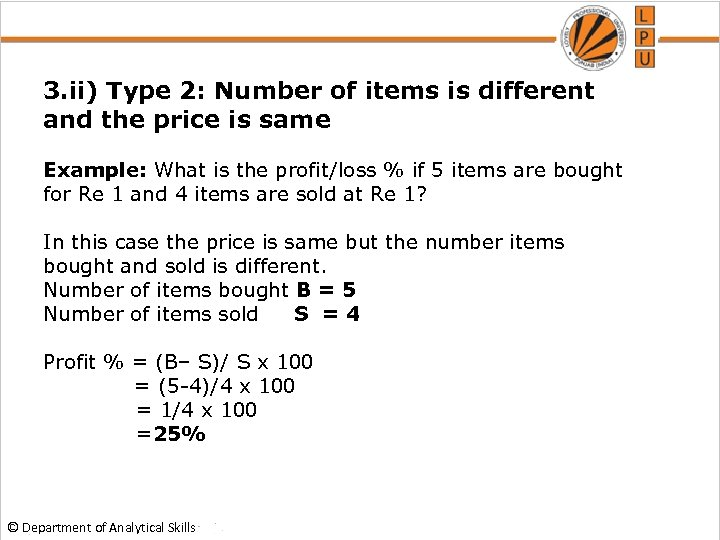3. ii) Type 2: Number of items is different and the price is same Example: What is the profit/loss % if 5 items are bought for Re 1 and 4 items are sold at Re 1? In this case the price is same but the number items bought and sold is different. Number of items bought B = 5 Number of items sold S =4 Profit % = (B– S)/ S x 100 = (5 -4)/4 x 100 = 1/4 x 100 =25% © Department of Analytical Skills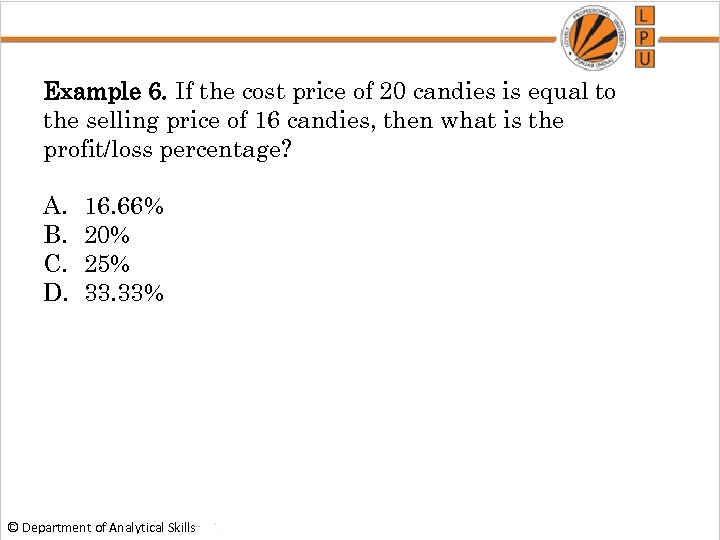Example 6. If the cost price of 20 candies is equal to the selling price of 16 candies, then what is the profit/loss percentage? A. B. C. D. 16. 66% 20% 25% 33. 33% © Department of Analytical Skills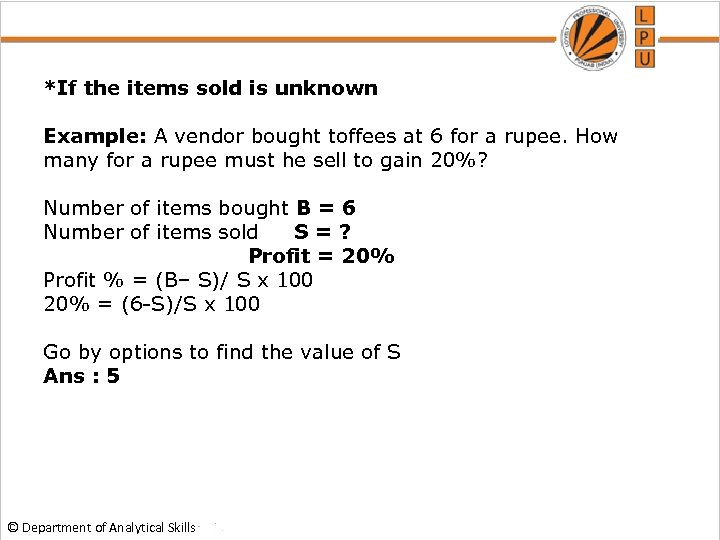*If the items sold is unknown Example: A vendor bought toffees at 6 for a rupee. How many for a rupee must he sell to gain 20%? Number of items bought B = 6 Number of items sold S=? Profit = 20% Profit % = (B– S)/ S x 100 20% = (6 -S)/S x 100 Go by options to find the value of S Ans : 5 © Department of Analytical Skills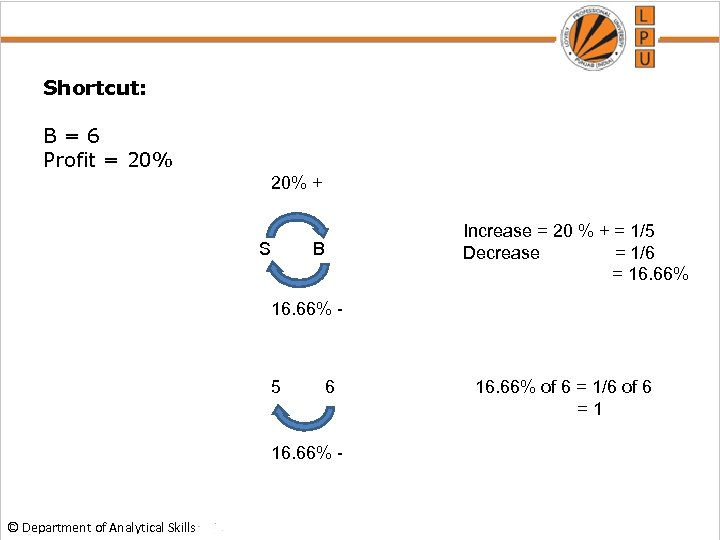Shortcut: B=6 Profit = 20% + S Increase = 20 % + = 1/5 Decrease = 1/6 = 16. 66% B 16. 66% - 5 6 16. 66% - © Department of Analytical Skills 16. 66% of 6 = 1/6 of 6 =1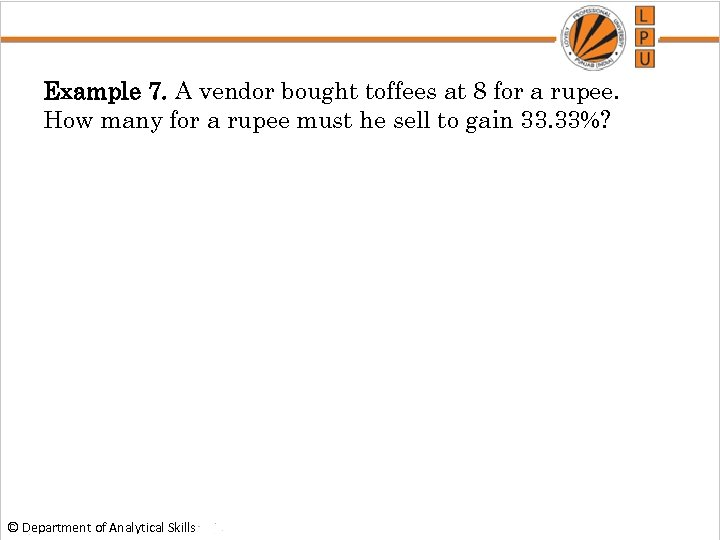Example 7. A vendor bought toffees at 8 for a rupee. How many for a rupee must he sell to gain 33. 33%? © Department of Analytical Skills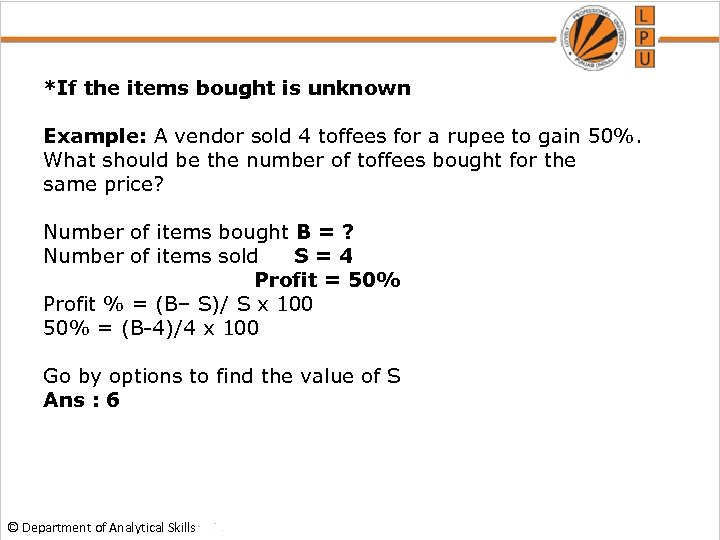*If the items bought is unknown Example: A vendor sold 4 toffees for a rupee to gain 50%. What should be the number of toffees bought for the same price? Number of items bought B = ? Number of items sold S=4 Profit = 50% Profit % = (B– S)/ S x 100 50% = (B-4)/4 x 100 Go by options to find the value of S Ans : 6 © Department of Analytical Skills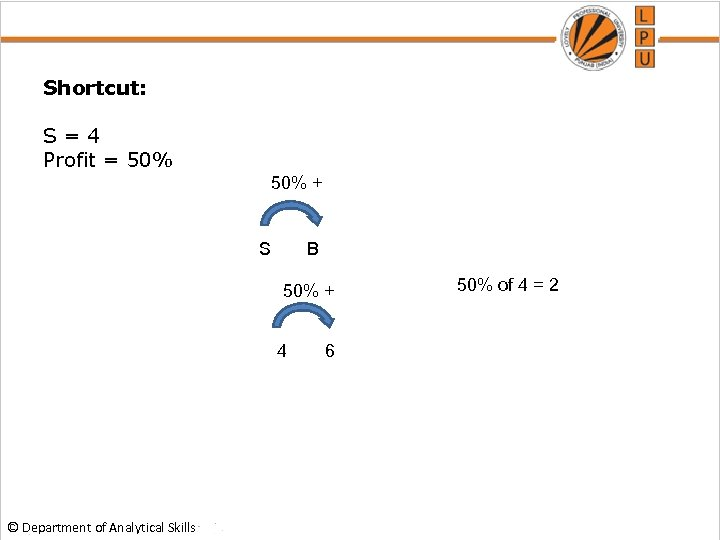Shortcut: S=4 Profit = 50% + S B 50% + 4 © Department of Analytical Skills 6 50% of 4 = 2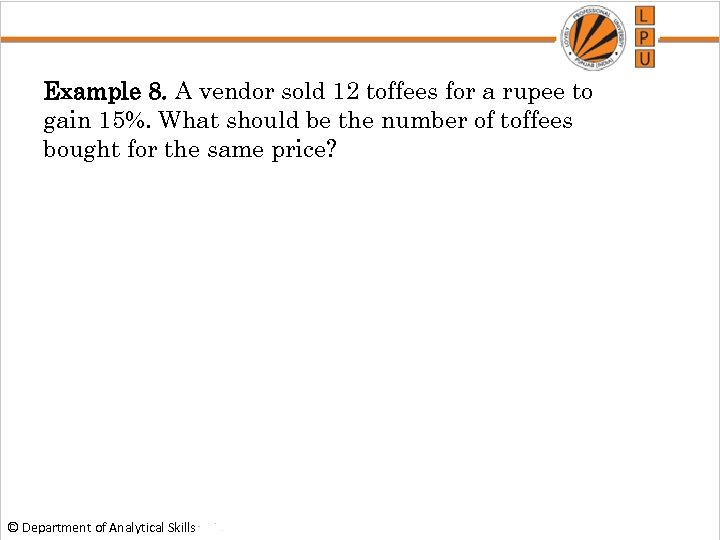Example 8. A vendor sold 12 toffees for a rupee to gain 15%. What should be the number of toffees bought for the same price? © Department of Analytical Skills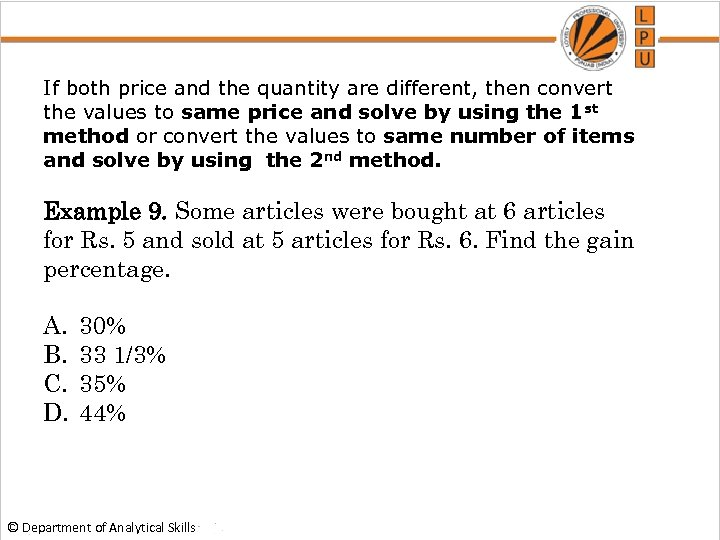If both price and the quantity are different, then convert the values to same price and solve by using the 1 st method or convert the values to same number of items and solve by using the 2 nd method. Example 9. Some articles were bought at 6 articles for Rs. 5 and sold at 5 articles for Rs. 6. Find the gain percentage. A. B. C. D. 30% 33 1/3% 35% 44% © Department of Analytical Skills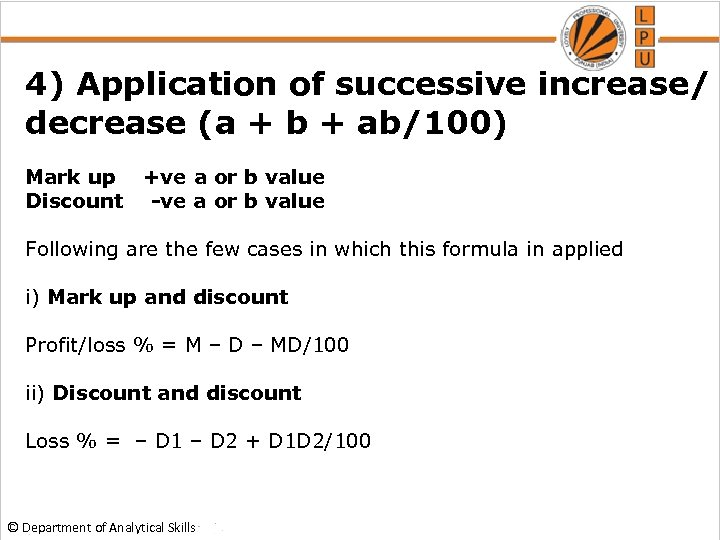4) Application of successive increase/ decrease (a + b + ab/100) Mark up +ve a or b value Discount -ve a or b value Following are the few cases in which this formula in applied i) Mark up and discount Profit/loss % = M – D – MD/100 ii) Discount and discount Loss % = – D 1 – D 2 + D 1 D 2/100 © Department of Analytical Skills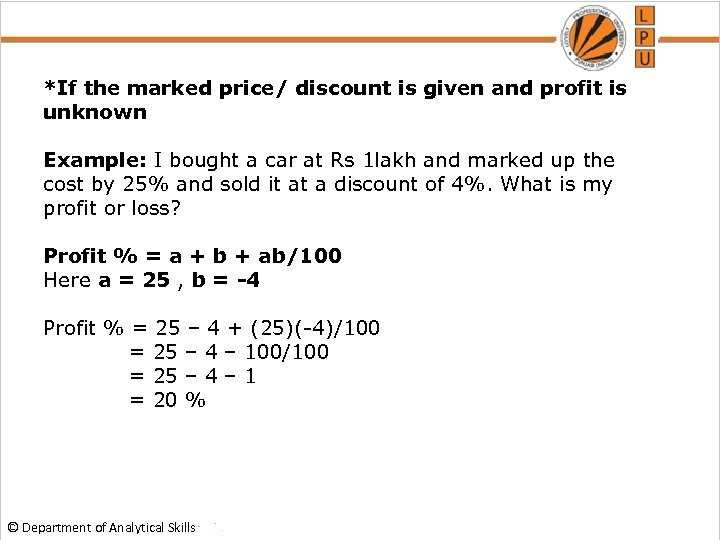*If the marked price/ discount is given and profit is unknown Example: I bought a car at Rs 1 lakh and marked up the cost by 25% and sold it at a discount of 4%. What is my profit or loss? Profit % = a + b + ab/100 Here a = 25 , b = -4 Profit % = 25 – 4 + (25)(-4)/100 = 25 – 4 – 100/100 = 25 – 4 – 1 = 20 % © Department of Analytical Skills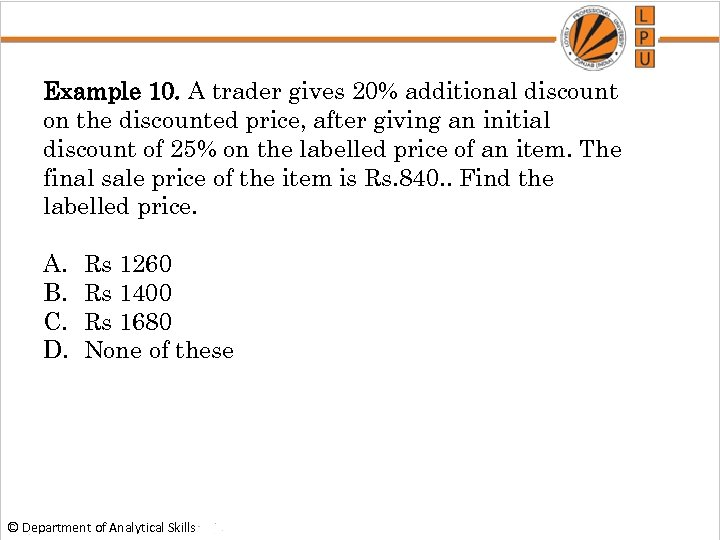Example 10. A trader gives 20% additional discount on the discounted price, after giving an initial discount of 25% on the labelled price of an item. The final sale price of the item is Rs. 840. . Find the labelled price. A. B. C. D. Rs 1260 Rs 1400 Rs 1680 None of these © Department of Analytical Skills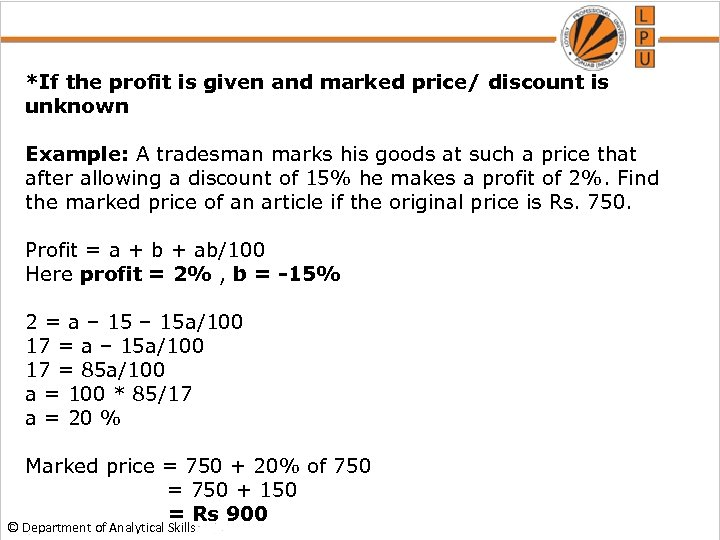*If the profit is given and marked price/ discount is unknown Example: A tradesman marks his goods at such a price that after allowing a discount of 15% he makes a profit of 2%. Find the marked price of an article if the original price is Rs. 750. Profit = a + b + ab/100 Here profit = 2% , b = -15% 2 = a – 15 a/100 17 = 85 a/100 a = 100 * 85/17 a = 20 % Marked price = 750 + 20% of 750 = 750 + 150 = Rs 900 © Department of Analytical Skills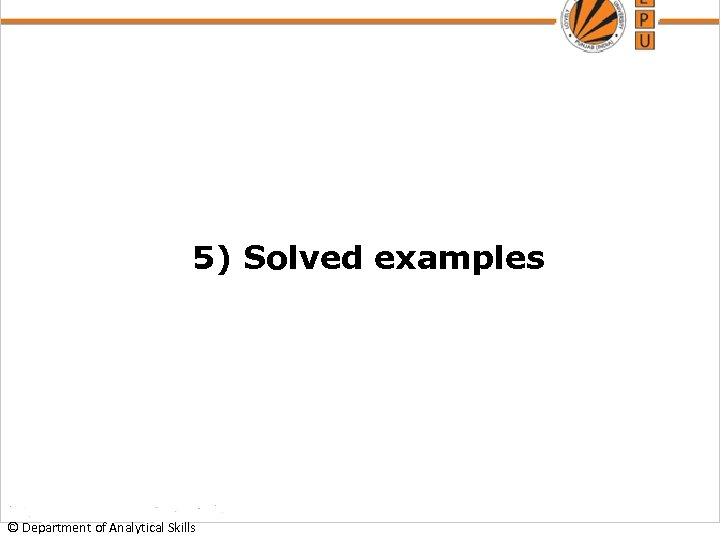5) Solved examples © Department of Analytical Skills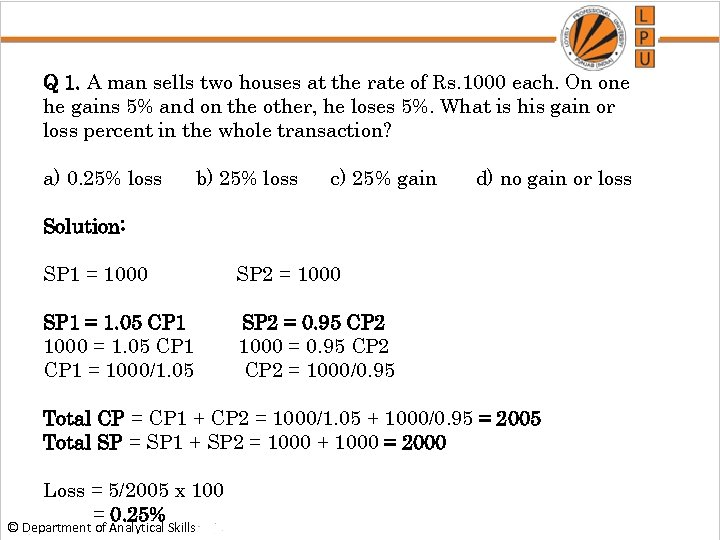Q 1. A man sells two houses at the rate of Rs. 1000 each. On one he gains 5% and on the other, he loses 5%. What is his gain or loss percent in the whole transaction? a) 0. 25% loss b) 25% loss c) 25% gain d) no gain or loss Solution: SP 1 = 1000 SP 2 = 1000 SP 1 = 1. 05 CP 1 1000 = 1. 05 CP 1 = 1000/1. 05 SP 2 = 0. 95 CP 2 1000 = 0. 95 CP 2 = 1000/0. 95 Total CP = CP 1 + CP 2 = 1000/1. 05 + 1000/0. 95 = 2005 Total SP = SP 1 + SP 2 = 1000 + 1000 = 2000 Loss = 5/2005 x 100 = 0. 25% © Department of Analytical Skills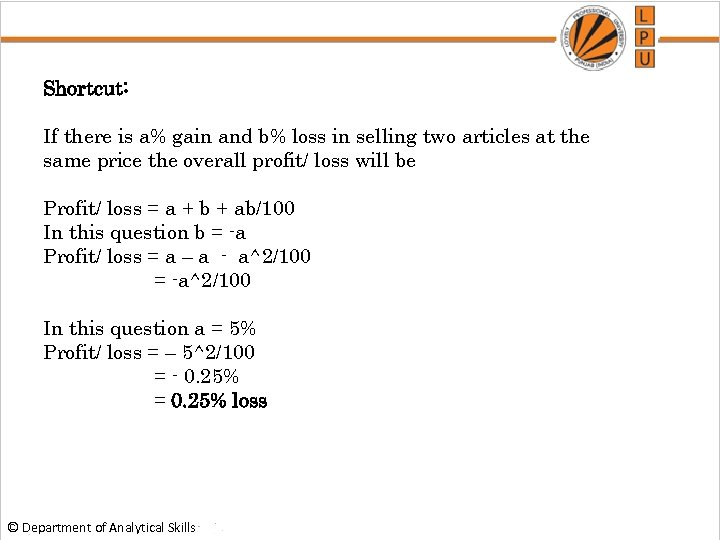Shortcut: If there is a% gain and b% loss in selling two articles at the same price the overall profit/ loss will be Profit/ loss = a + b + ab/100 In this question b = -a Profit/ loss = a – a - a^2/100 = -a^2/100 In this question a = 5% Profit/ loss = – 5^2/100 = - 0. 25% = 0. 25% loss © Department of Analytical Skills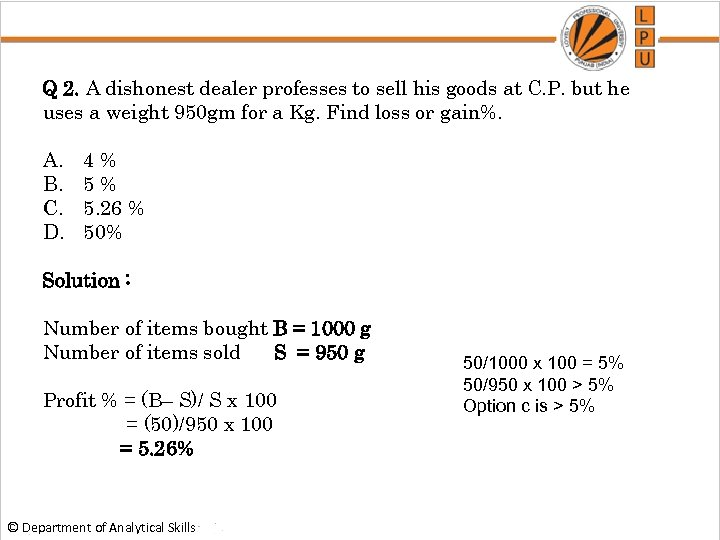Q 2. A dishonest dealer professes to sell his goods at C. P. but he uses a weight 950 gm for a Kg. Find loss or gain%. A. B. C. D. 4% 5% 5. 26 % 50% Solution : Number of items bought B = 1000 g Number of items sold S = 950 g Profit % = (B– S)/ S x 100 = (50)/950 x 100 = 5. 26% © Department of Analytical Skills 50/1000 x 100 = 5% 50/950 x 100 > 5% Option c is > 5%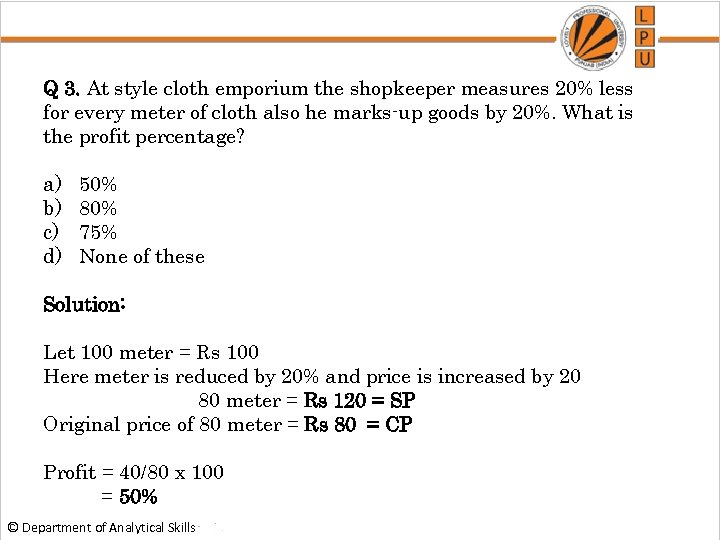Q 3. At style cloth emporium the shopkeeper measures 20% less for every meter of cloth also he marks-up goods by 20%. What is the profit percentage? a) b) c) d) 50% 80% 75% None of these Solution: Let 100 meter = Rs 100 Here meter is reduced by 20% and price is increased by 20 80 meter = Rs 120 = SP Original price of 80 meter = Rs 80 = CP Profit = 40/80 x 100 = 50% © Department of Analytical Skills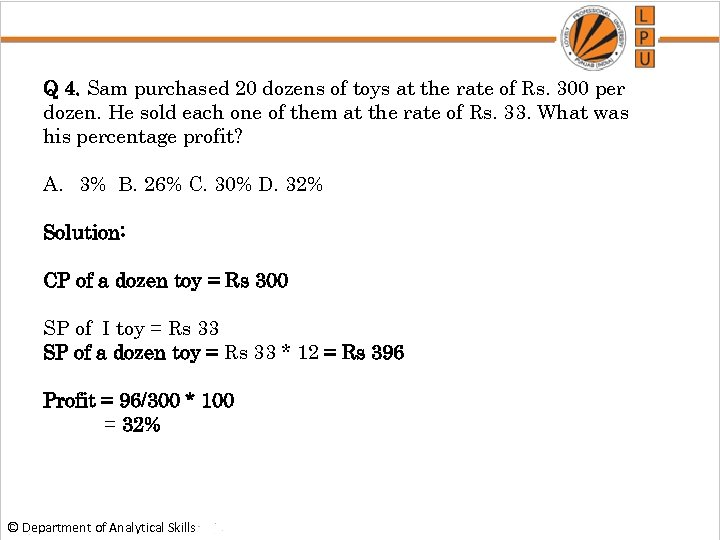Q 4. Sam purchased 20 dozens of toys at the rate of Rs. 300 per dozen. He sold each one of them at the rate of Rs. 33. What was his percentage profit? A. 3% B. 26% C. 30% D. 32% Solution: CP of a dozen toy = Rs 300 SP of I toy = Rs 33 SP of a dozen toy = Rs 33 * 12 = Rs 396 Profit = 96/300 * 100 = 32% © Department of Analytical Skills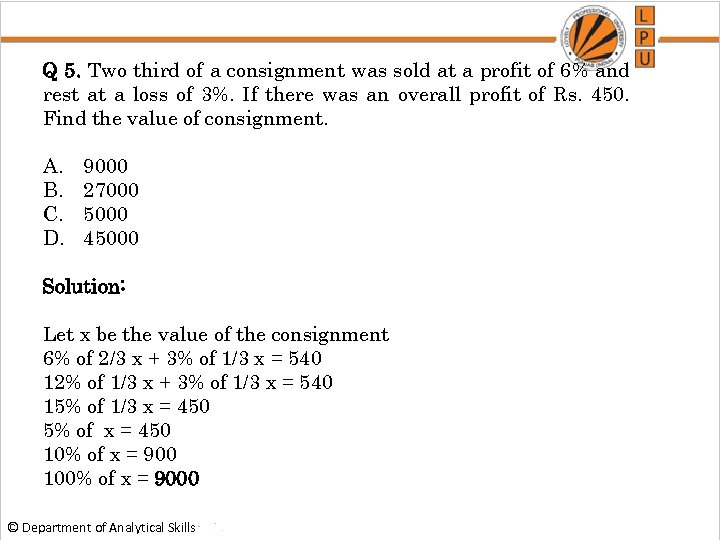Q 5. Two third of a consignment was sold at a profit of 6% and rest at a loss of 3%. If there was an overall profit of Rs. 450. Find the value of consignment. A. B. C. D. 9000 27000 5000 45000 Solution: Let x be the value of the consignment 6% of 2/3 x + 3% of 1/3 x = 540 12% of 1/3 x + 3% of 1/3 x = 540 15% of 1/3 x = 450 5% of x = 450 10% of x = 900 100% of x = 9000 © Department of Analytical Skills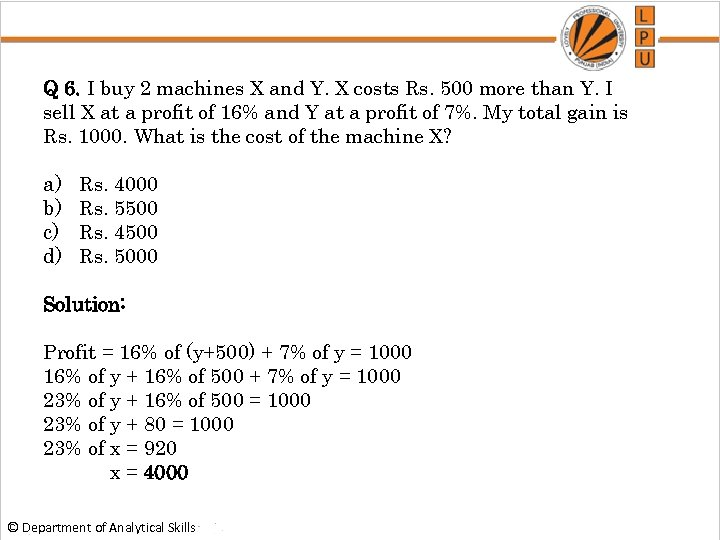Q 6. I buy 2 machines X and Y. X costs Rs. 500 more than Y. I sell X at a profit of 16% and Y at a profit of 7%. My total gain is Rs. 1000. What is the cost of the machine X? a) b) c) d) Rs. 4000 Rs. 5500 Rs. 4500 Rs. 5000 Solution: Profit = 16% of (y+500) + 7% of y = 1000 16% of y + 16% of 500 + 7% of y = 1000 23% of y + 16% of 500 = 1000 23% of y + 80 = 1000 23% of x = 920 x = 4000 © Department of Analytical Skills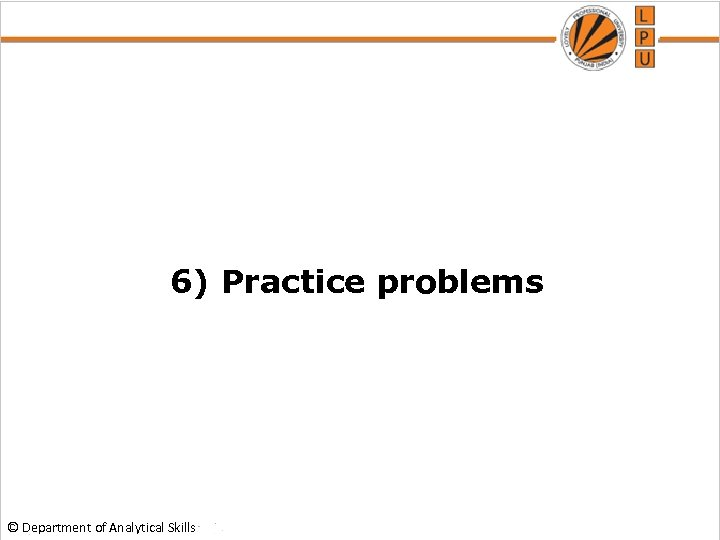6) Practice problems © Department of Analytical Skills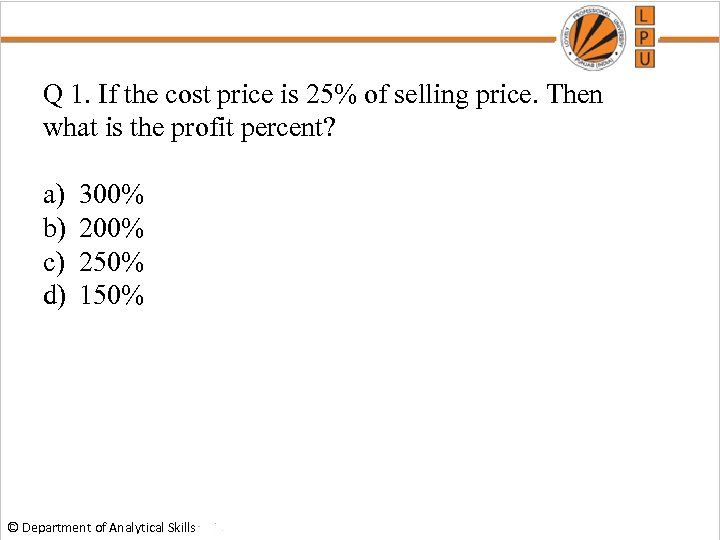Q 1. If the cost price is 25% of selling price. Then what is the profit percent? a) b) c) d) 300% 250% 150% © Department of Analytical Skills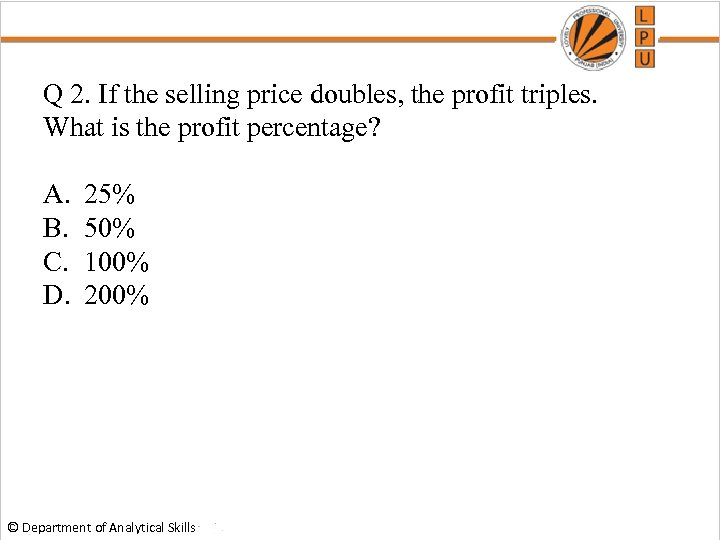Q 2. If the selling price doubles, the profit triples. What is the profit percentage? A. B. C. D. 25% 50% 100% 200% © Department of Analytical Skills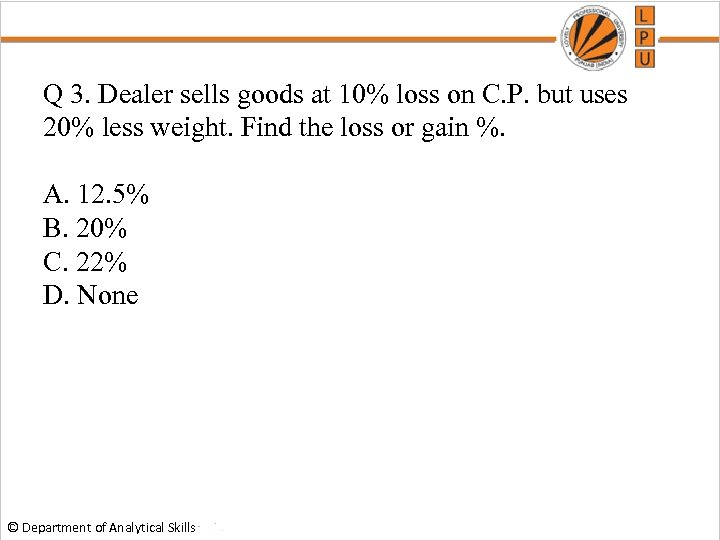Q 3. Dealer sells goods at 10% loss on C. P. but uses 20% less weight. Find the loss or gain %. A. 12. 5% B. 20% C. 22% D. None © Department of Analytical Skills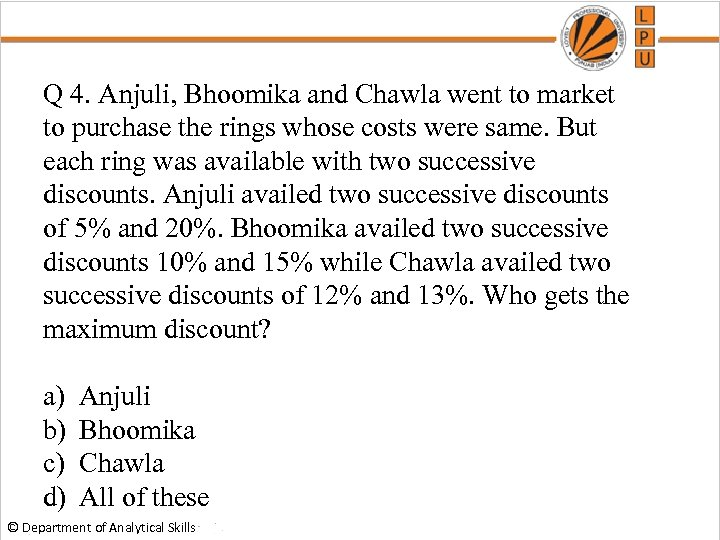Q 4. Anjuli, Bhoomika and Chawla went to market to purchase the rings whose costs were same. But each ring was available with two successive discounts. Anjuli availed two successive discounts of 5% and 20%. Bhoomika availed two successive discounts 10% and 15% while Chawla availed two successive discounts of 12% and 13%. Who gets the maximum discount? a) b) c) d) Anjuli Bhoomika Chawla All of these © Department of Analytical Skills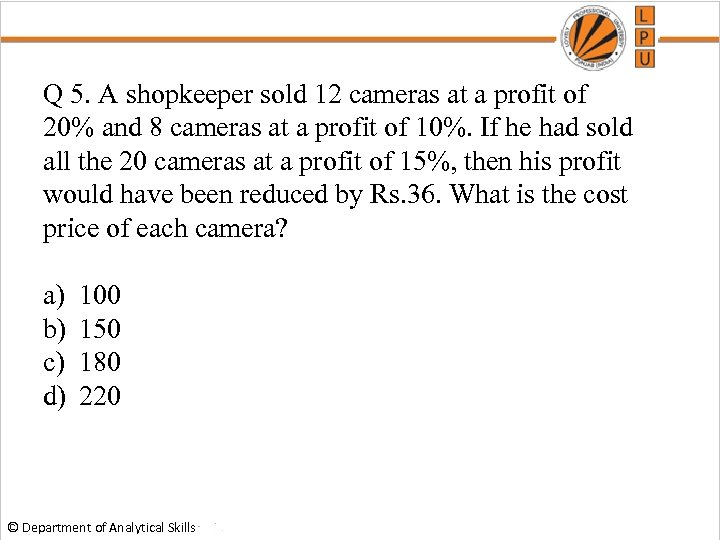Q 5. A shopkeeper sold 12 cameras at a profit of 20% and 8 cameras at a profit of 10%. If he had sold all the 20 cameras at a profit of 15%, then his profit would have been reduced by Rs. 36. What is the cost price of each camera? a) b) c) d) 100 150 180 220 © Department of Analytical Skills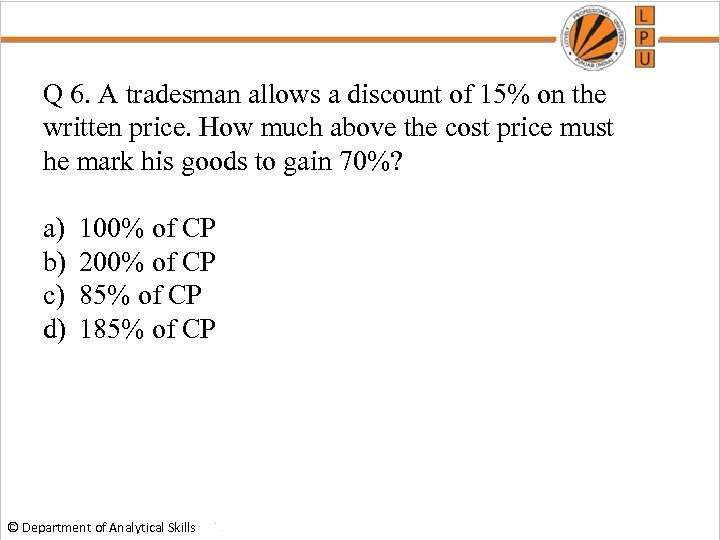Q 6. A tradesman allows a discount of 15% on the written price. How much above the cost price must he mark his goods to gain 70%? a) b) c) d) 100% of CP 200% of CP 85% of CP 185% of CP © Department of Analytical Skills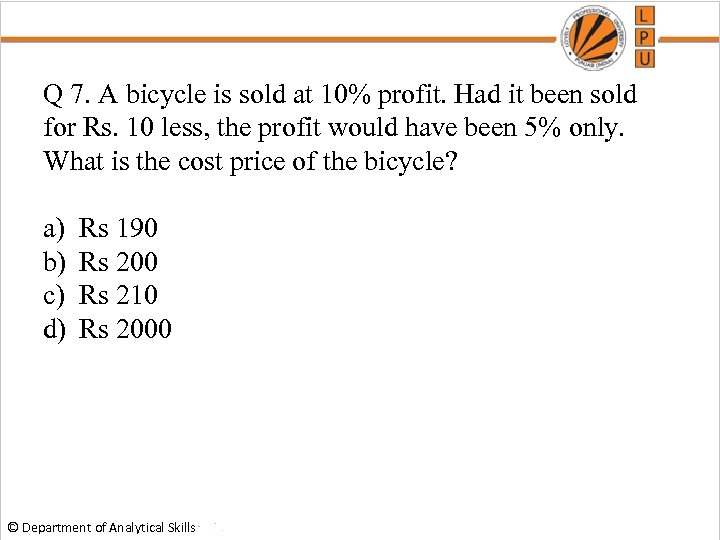Q 7. A bicycle is sold at 10% profit. Had it been sold for Rs. 10 less, the profit would have been 5% only. What is the cost price of the bicycle? a) b) c) d) Rs 190 Rs 200 Rs 210 Rs 2000 © Department of Analytical Skills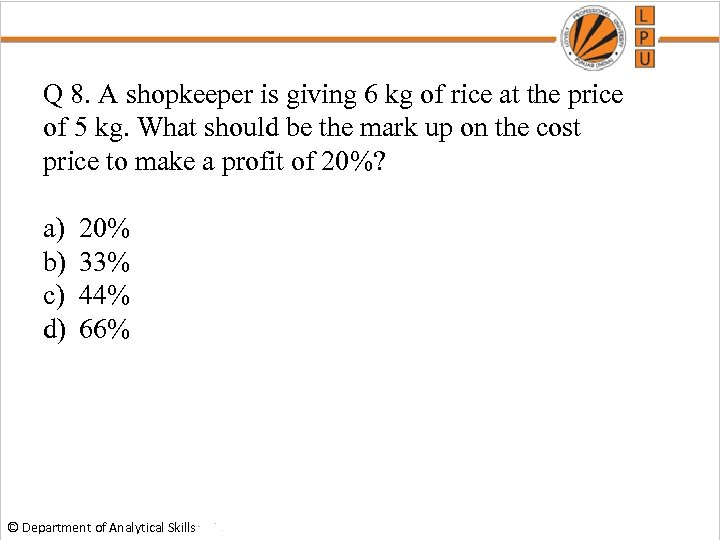Q 8. A shopkeeper is giving 6 kg of rice at the price of 5 kg. What should be the mark up on the cost price to make a profit of 20%? a) b) c) d) 20% 33% 44% 66% © Department of Analytical Skills